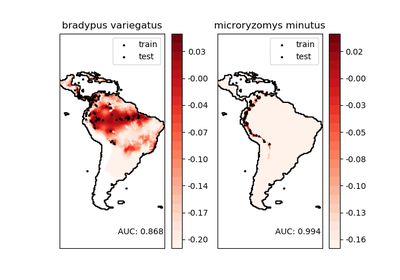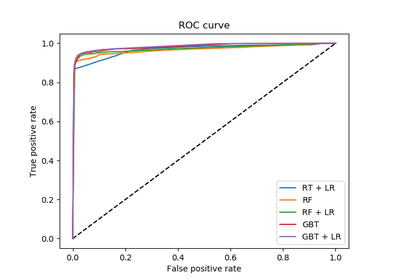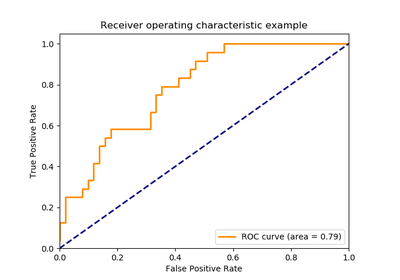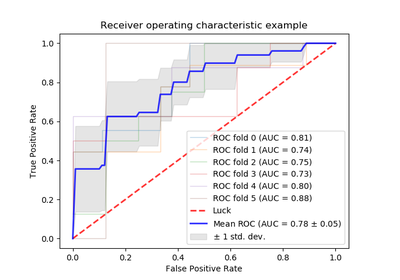# `sklearn.metrics`.roc_curve¶

`sklearn.metrics.``roc_curve`(y_true, y_score, pos_label=None, sample_weight=None, drop_intermediate=True)[source]

Note: this implementation is restricted to the binary classification task.

Read more in the User Guide.

Parameters: y_true : array, shape = [n_samples] True binary labels in range {0, 1} or {-1, 1}. If labels are not binary, pos_label should be explicitly given. y_score : array, shape = [n_samples] Target scores, can either be probability estimates of the positive class, confidence values, or non-thresholded measure of decisions (as returned by “decision_function” on some classifiers). pos_label : int or str, default=None Label considered as positive and others are considered negative. sample_weight : array-like of shape = [n_samples], optional Sample weights. drop_intermediate : boolean, optional (default=True) Whether to drop some suboptimal thresholds which would not appear on a plotted ROC curve. This is useful in order to create lighter ROC curves. New in version 0.17: parameter drop_intermediate. fpr : array, shape = [>2] Increasing false positive rates such that element i is the false positive rate of predictions with score >= thresholds[i]. tpr : array, shape = [>2] Increasing true positive rates such that element i is the true positive rate of predictions with score >= thresholds[i]. thresholds : array, shape = [n_thresholds] Decreasing thresholds on the decision function used to compute fpr and tpr. thresholds represents no instances being predicted and is arbitrarily set to max(y_score) + 1.

`roc_auc_score`
Compute the area under the ROC curve

Notes

Since the thresholds are sorted from low to high values, they are reversed upon returning them to ensure they correspond to both `fpr` and `tpr`, which are sorted in reversed order during their calculation.

References

Examples

```>>> import numpy as np
>>> from sklearn import metrics
>>> y = np.array([1, 1, 2, 2])
>>> scores = np.array([0.1, 0.4, 0.35, 0.8])
>>> fpr, tpr, thresholds = metrics.roc_curve(y, scores, pos_label=2)
>>> fpr
array([ 0. ,  0.5,  0.5,  1. ])
>>> tpr
array([ 0.5,  0.5,  1. ,  1. ])
>>> thresholds
array([ 0.8 ,  0.4 ,  0.35,  0.1 ])
```

## Examples using `sklearn.metrics.roc_curve`¶Species distribution modelingFeature transformations with ensembles of trees